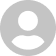Extended Plugin
3.6

5.0# Extended Plugin

3.6
15 April 2010

REALbasic plug-in adds new data types.

## Overview

Extended Plugin for REALbasic 5 and higher adds five new data types. They are:
• ExtInteger
• ExtFloat
• ExtComplex
• IAExtFloat
• ExtFraction

ExtInteger is the type ZZ of Victor Shoup\'s multi-precision library, NTL. Except for available memory, there is no limitation on the size of an ExtInteger. So you can multiply a 100 digit integer by another 100 digit integer giving the exact 200 digit integer.

ExtFloat is the type RR of NTL. You can set both the bit precision and the decimal output precision for ExtFloat, with no limitation except for available memory. It might take awhile, but you can now calculate pi in a REALbasic program to a million decimal places, or more. The power of 10 for ExtFloat can range from about -60,000,000 to +60,000,000 as compared to -308 to +308 for a Double.

ExtComplex doesn\'t exist in NTL. I made it from two ExtFloats. So the real and imaginary parts of an ExtComplex use the bit precision and the decimal output precision set for ExtFloat. So you can now use complex numbers with almost unlimited precision.

IAExtFloat also doesn\'t exist in NTL. It is a data type which is used for Interval Arithmetic, which is arithmetic with inequalities. An IAExtFloat number is represented by two ExtFloat numbers (a, b), where one thinks of an ExtFloat number x being in the interval between a and b. That is: a <= x <= b. For a function of x calculated with interval arithmetic the result is an IAExtFloat whose interval (fa, fb) results from interval propagation.

ExtFraction handles a fraction as num/den, where num and den are ExtIntegers. Thus one can do exact calculations with rationals in REALbasic to an almost unlimited degree.

## What's new in Extended Plugin

Version 3.6:
• adds support for Mac Cocoa.

#### Join over 500,000 subscribers.

Subscribe for our newsletter with best Mac offers from MacUpdate.

## 1 Extended Plugin Reviews

Rate this app:15 April 2010